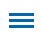MENUThe Common Core State Standards for Mathematics begin with eight Standards for Mathematical Practice. These aren't content standards, but behaviors, expertise, and habits of mind necessary for successful work with math. Great math instruction pairs content standards with these standards of practice to ensure student success with math.

In preparing for an upcoming workshop on Implementing the Standards for Mathematical Practice, I was trying to find some specific practical actions to help educators successfully instill these habits of mind in their students.While teacher modeling, task selection, and discussions are crucial to success in these areas, I found myself circling back to questioning strategies again and again. (Too much time with Bloom's?)

Here are examples of the types of questions you can ask to build student expertise in the area of focus for each Standard for Mathematical Practice.

## 1. Make sense of problems and persevere in solving them.

• Before we move on, are we sure we understand this?
• What are our options?
• Do we have a plan?
• Are we making progress or should we reconsider?
• Why do we think this is true?

## 2. Reason abstractly and quantitatively.

• What if...?
• How else can we...?
• Does this always work? Why?

## 3. Construct viable arguments and critique the reasoning of others.

• Why do you think it's true?
• What is an acceptable argument?
• Does this always work?

## 4. Model with mathematics.

• How could we break this down?
• What does this remind you of?
• What do the numbers show?
• How are the values similar? Different?

## 5. Use appropriate tools strategically.

• What can we use to...?
• What else can we use to...?
• What would work the best?
• What can't we do with this?

## 6. Attend to precision.

• Should we recheck our work?
• Is it appropriate to estimate?
• Is this quality work?

## 7. Look for and make use of structure.

• What is happening here?
• What goes together?
• What can we do first?
• Can we break it down? How?

## 8. Look for and express regularity in repeated reasoning.

• Can you describe what is happening?
• How is that the same? Different?
• How is that different?
• Where did we see that before?
• What do you think will happen next?

While student expertise with math still requires deep understanding of the math
content standards, building these behaviors and habits of mind help provide them
with the disposition to "see themselves as capable of learning mathematics and using
it to solve problems." (Adding It Up: Helping Children Learn Mathematics)

Asking questions might just be the best modeling you can do, since the ability to ask effective questions is essential to success with math.